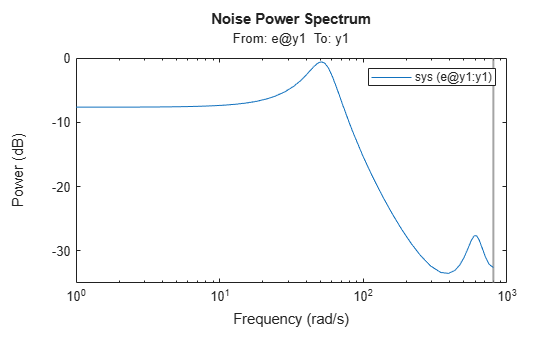# Analyze Time-Series Models

This example shows how to analyze time-series models.

A time-series model has no inputs. However, you can use many response computation commands on such models. The software treats (implicitly) the noise source `e(t)` as a measured input. Thus, `step(sys)` plots the step response assuming that the step input was applied to the noise channel `e(t)`.

To avoid ambiguity in how the software treats a time-series model, you can transform it explicitly into an input-output model using `noise2meas`. This command causes the noise input `e(t)` to be treated as a measured input and transforms the linear time series model with `Ny` outputs into an input-output model with `Ny` outputs and `Ny` inputs. You can use the resulting model with commands, such as, `bode`, `nyquist`, and `iopzmap` to study the characteristics of the `H` transfer function.

Estimate a time-series model.

```load iddata9 sys = ar(z9,4);```

Convert the time-series model to an input-output model.

`iosys = noise2meas(sys);`

Plot the step response of `H`.

`step(iosys);`Plot the poles and zeros of `H`.

`iopzmap(iosys);`Calculate and plot the time-series spectrum directly without converting to an input-output model.

`spectrum(sys);`The command plots the time-series spectrum amplitude $\Phi \left(\omega \right)={‖H\left(\omega \right)‖}^{2}$.